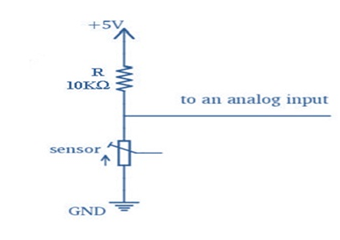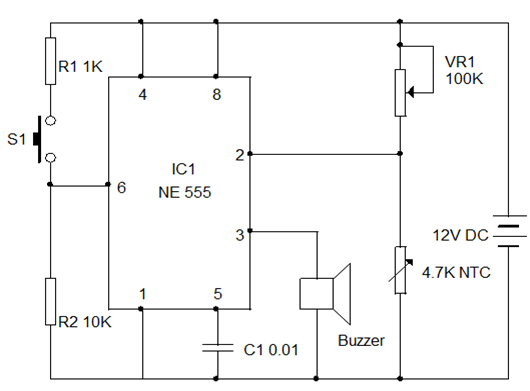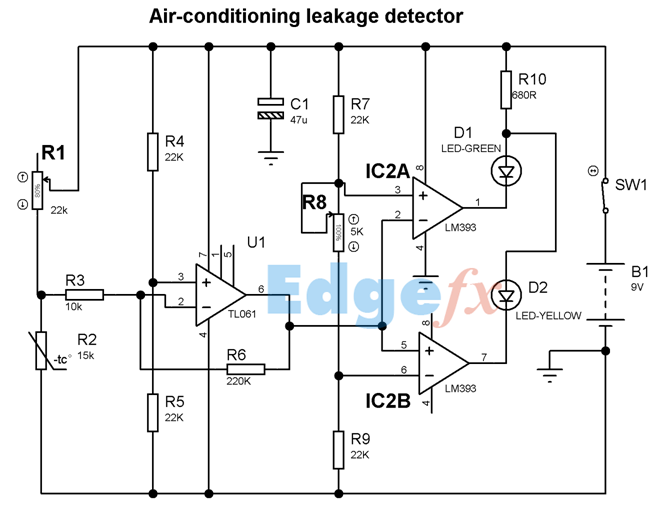# Temperature Sensors Applications

Here we have two practical applications involving circuits to sense the temperature using sensors and gives an electrical output. In both the circuits, we have used an analog circuit. So let us have a brief idea about analog circuits.

A sensor is a unit that can measure a physical phenomenon and quantify the latter, in other words it gives a measurable representation of the wonder on a particular scale or range. Generally sensors are categorized into two types, analog and digital sensors. Here we are going to discuss about the analog sensor.

An analog sensor is a component than measures any actual magnitude and translates its value into a magnitude that we can measure with an electronic circuit, ordinarily a resistor or capacitive value that we can change over into a voltage quality. Example of an analog sensor could be a thermistor, where resistor changes its resistance based on the temperature. Most of the analog sensors usually come with three connection pins, one for getting supply voltage, one for ground association and last one is the output voltage pin. Most of the analog sensors we are going to use are resistive sensors, is shown in figure. It is wired into a circuit in a manner that it will have an output with a particular voltage range; generally voltage range is between 0 volts to 5 volts. Finally we can get this value into our microcontroller using one of its analog input pin. Analog sensors measure door position, water, power and smoke of devices.

###1. A Simple Heat Sensor

Make this simple heat sensor circuit to monitor the temperature in heat generating devices like Amplifier and Inverter. When the temperature in the device exceeds the allowable limit, the circuit warns through beeps. It is too simple and can be fixed in the device itself with the power tapped from it. The circuit works in 5 to 12 volts DC.

The circuit is designed using the popular timer IC 555 in the Bistable mode. IC 555 has two comparators, a flip flop and an output stage. Its output becomes high when a negative pulse more than 1/3 Vcc is applied to its trigger pin 2. At this time, the lower comparator triggers and changes the state of the flip-flop and the output turns high. That is, if the voltage at pin 2 is less than 1/3 Vcc, output goes high and if it is higher than 1/3 Vcc, the output remains low.

Here an NTC (Negative Temperature Coefficient) Thermister is used as the heat sensor. It is a kind of variable resistor and its resistance depends on the temperature around it. In NTC Thermister, the resistance drops when the temperature in its vicinity increases. But in PTC (Positive Temperature Coefficient) Thermistor, the resistance increases when the temperature increases.

In the circuit, the 4.7K NTC Thermistor is connected to pin2 of IC1. Variable resistor VR1 adjusts the sensitivity of the Thermistor at the particular temperature level. In order to reset the flip-flop and hence to change the output, the threshold pin 6 of IC1 is used. When a positive pulse is applied to pin 6 through the push switch, the upper comparator of IC1 becomes high and triggers the R input of the flip-flop. This resets and the output turns low.When the temperature of the device is normal (as set by VR1), output of IC1 remains low because the trigger pin 2 is getting more than 1/3 Vcc. This keeps the output low and the buzzer remains silent. When the temperature in the device increases due to prolonged use or any short in the power supply, resistance of the Thermister decreases taking the trigger pin less than 1/3 Vcc. The Bistable then triggers and its output goes high. This activates the buzzer and beeps will be generated. This state continues till the temperature decreases or IC resets by pressing S1.

### How to Set?

Assemble the circuit on a common PCB and fix inside the device to be monitored. Connect the Thermister (the Thermister has no polarity) with the circuit using thin wires. Fix the Thermister near the heat generating parts of the device like, transformer or heat sink. Power can be tapped from the power supply of the device. Power the circuit and switch on the device. Slowly adjust VR1 till the buzzer stops at normal temperature. The circuit will become active when the temperature inside the device rises.

### 2. Air Conditioning Leakage Detector

It is a comparator which detects temperature changes with respect to the surrounding temperature. It was primarily intended to detect droughts around doors and windows that cause energy leaks but can be used in many other ways, when a sensitive temperature change detector is needed. If the temperature change is pointing above, the red LED glows and if the temperature change points below, green LED glows.

Air Conditioning Leakage Detector Circuit DiagramHere, IC1 is used as a bridge detector and amplifier whose output voltage increases when temperature rises because of the bridge unbalancing. The 2 other ICs are used as comparator. Both the LEDs are off by varying R1 to balance the bridge. When the bridge is unbalanced due to change in temperature, one of LED will be illuminated.

Parts:

R1 = 22K – Linear Potentiometer

R2 = 15K @ 20°C n.t.c. Thermistor (See Notes)

R3 = 10K – 1/4W Resistor

R4 = 22K – 1/4W Resistor

R5 = 22K – 1/4W Resistor

R6 = 220K – 1/4W Resistor

R7 = 22K – 1/4W Resistor

R8 = 5K – preset

R9 = 22K – 1/4W Resistor

R10 = 680R – 1/4W Resistor

C1 = 47µF , 63V Electrolytic Capacitor

D1 = 5mm. LED Green

D2 = 5mm. LED  Yellow /White

U1 = TL061 IC ,Low current BIFET Op-Amp

IC2 = LM393 Dual Voltage Comparator IC

P1 = SPST Switch

B1 = 9V PP3 Battery

Notes:

• The resistance range of thermistors should be 10 to 20K in the 20 degrees range.
• The value of R1 should be twice the value of thermistor resistance.
• Thermistor should be enclosed in a small casing to ensure fast detection of temperature changes.
• Pin1 of IC2B should be connected to pin7 of IC2A if only one LED is needed.